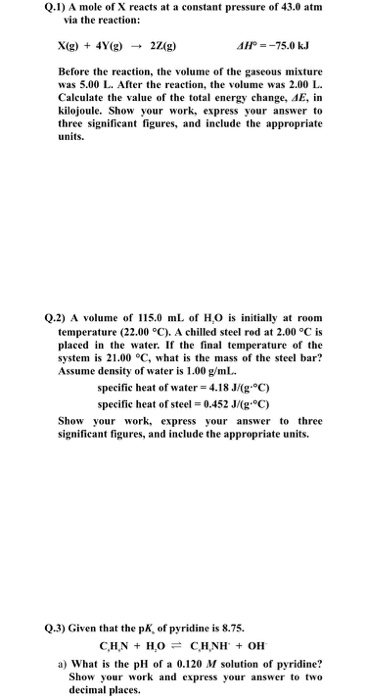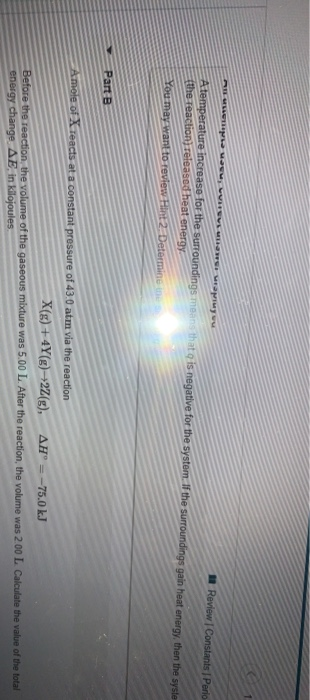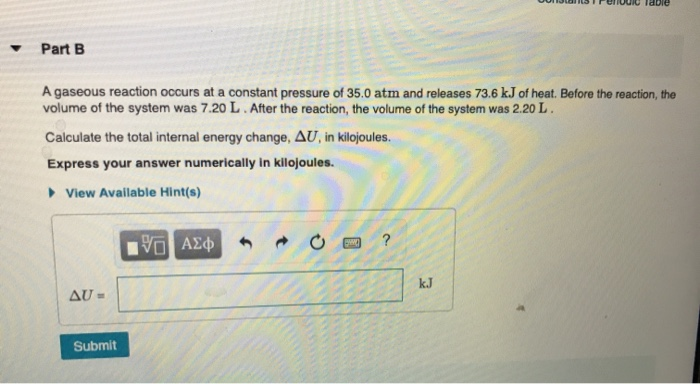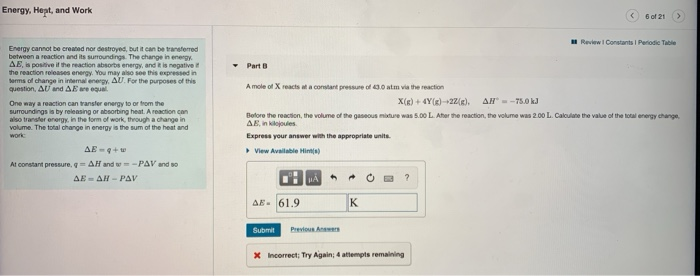Question

# A mole of X reacts at a constant pressure of 43.0 atm via the reaction X(g)+4Y(g)→2Z(g),    &nbs...

A mole of X reacts at a constant pressure of 43.0 atm via the reaction

X(g)+4Y(g)→2Z(g),      ΔH∘=−75.0 kJ

Before the reaction, the volume of the gaseous mixture was 5.00 L. After the reaction, the volume was 2.00 L. Calculate the value of the total energy change, ΔE, in kilojoules.

The following quantities are given

ΔH0 = -75.0 kJ , Pressure, P = 43.0 atm = 43 atm * 101325 Pa/1 atm = 4356975 Pa = 4356975 N / m2

Volume change, ΔV = Final - Initial = 2.0 L - 5.0 L = -3.0 L = -3.0 *10-3 m3

Work done, w = - PΔV
= - (4356975 N / m2) (-3.0 *10-3 m3)
= 13070 N m
= 13070 J
= 13.07 kJ
We know that at consatnt pressure qp = ΔH0 = -75.0 kJ
Also ΔE = q + w
= - 75.0 kJ + 13.07 kJ
= -61.93 kJ

#### Earn Coins

Coins can be redeemed for fabulous gifts.

Similar Homework Help Questions
• ### A mole of X reacts at a constant pressure of 43.0 atm via the reaction X(g)+4Y(g)→2Z(g), ΔH∘=−75.0 kJ Before the reacti...

A mole of X reacts at a constant pressure of 43.0 atm via the reaction X(g)+4Y(g)→2Z(g), ΔH∘=−75.0 kJ Before the reaction, the volume of the gaseous mixture was 5.00 L. After the reaction, the volume was 2.00 L. Calculate the value of the total energy change, ΔE, in kilojoules.

• ### Assuming constant pressure, rank these reactions from most energy released by the system to most energy absorbed by the...

Assuming constant pressure, rank these reactions from most energy released by the system to most energy absorbed by the system, based on the following descriptions: Surroundings get colder and the system decreases in volume. Surroundings get hotter and the system expands in volume. Surroundings get hotter and the system decreases in volume. Surroundings get hotter and the system does not change in volume. Also assume that the magnitude of the volume and temperature changes are similar among the reactions. Rank...

• ### please solve this on paper wiyh the steps thank u Q.1) A mole of X reacts...please solve this on paper wiyh the steps thank u Q.1) A mole of X reacts at a constant pressure of 43.0 atm via the reaction: X(g) + 4Y(g) + 2/(g) AH° = -75.0 kJ Before the reaction, the volume of the gaseous mixture was 5.00 L. After the reaction, the volume was 2.00 L. Calculate the value of the total energy change, AE, in kilojoule. Show your work, express your answer to three significant figures, and include the appropriate...

• ### Review Constants Perio A temperature increase for the surroundings mo the reactions released heat energy t...Review Constants Perio A temperature increase for the surroundings mo the reactions released heat energy t s negative for the system. I the surroundings gain heat energy, then the syste You may want to review int Determine Part B Amole of Teacts at a constant pressure of 43.0 atm via the reaction X(g) + 4Y()+22(g), AH = -75.0 kJ Before the reaction, the volume of the gaseous mixture was 5.00 L. After the reaction, the volume was 200 L Calculate...

• ### DIFCIU idue Part B A gaseous reaction occurs at a constant pressure of 35.0 atm and...DIFCIU idue Part B A gaseous reaction occurs at a constant pressure of 35.0 atm and releases 73.6 kJ of heat. Before the reaction, the volume of the system was 7.20 L. After the reaction, the volume of the system was 2.20 L. Calculate the total internal energy change, AU, in kilojoules. Express your answer numerically in kilojoules. View Available Hint(s) YO AE AU- Submit

• ### part a. The air in an inflated balloon (defined as the system) is warmed over a toaster and absorbs 110 J of heat. As it...

part a. The air in an inflated balloon (defined as the system) is warmed over a toaster and absorbs 110 J of heat. As it expands, it does 79 kJ of work. What is the change in internal energy for the system? Express the energy in kilojoules to two significant figures. part b. When fuel is burned in a cylinder equipped with a piston, the volume expands from 0.235 L to 1.350 L against an external pressure of 1.02 atm...

• ### An ideal gaseous reaction (which is a hypothetical gaseous reaction that conforms to the laws governing gas behavior) oc...

An ideal gaseous reaction (which is a hypothetical gaseous reaction that conforms to the laws governing gas behavior) occurs at a constant pressure of 50.0 atm and releases 65.0 kJ of heat. Before the reaction, the volume of the system was 7.60 L . After the reaction, the volume of the system was 2.20 L . Calculate the total internal energy change, ΔE, in kilojoules. Express your answer with the appropriate units.

• ### Part A A calorimeter contains 34.0 mL of water at 12.5 ∘C . When 1.50 g...

Part A A calorimeter contains 34.0 mL of water at 12.5 ∘C . When 1.50 g of X (a substance with a molar mass of 75.0 g/mol ) is added, it dissolves via the reaction X(s)+H2O(l)→X(aq) and the temperature of the solution increases to 25.5 ∘C . Calculate the enthalpy change, ΔH, for this reaction per mole of X. Assume that the specific heat of the resulting solution is equal to that of water [4.18 J/(g⋅∘C)], that density of water...

• ### Energy, Hept, and Work 621 > Review Constants Periodic Table Energy cannot be created nor destroyed, but it can...Energy, Hept, and Work 621 > Review Constants Periodic Table Energy cannot be created nor destroyed, but it can be transferred between a reaction and its surroundings. The change in energy AE is positive if the reaction absorbs energy, and it is negative the reaction release energy. You may also see this expressed in Dorms of change in internal energy. AU. For the purposes of this question, AU and we gua. One way a reaction can transfer energy to or...

• ### When 20.00 moles of H2(g) reacts with 10.00 mol of O2(g) to form 20.00 mol of...

When 20.00 moles of H2(g) reacts with 10.00 mol of O2(g) to form 20.00 mol of H2O(l) at 25°C and a constant pressure of 1.00 atm. If 1366 kJ of heat are released during this reaction, and PΔV is equal to -74.00 kJ, then ΔH° = -1366 kJ and ΔE° = -1292 kJ.ΔH° = +1366 kJ and ΔE° = +1440 kJ.ΔH° = -1366 kJ and ΔE° = -1440 kJ.ΔH° = +1366 kJ and ΔE° = +1292 kJ.